• 奇异值（Singular Value）往往对应着矩阵中的隐含的重要信息，且重要性与奇异值大小呈正相关。 关于奇异值的知识，可以...在图像处理中，奇异值小的部分往往代表着噪声，因此可以借助SVD算法来实现去噪。 选取
奇异值（Singular Value）往往对应着矩阵中的隐含的重要信息，且重要性与奇异值大小呈正相关。
关于奇异值的知识，可以参考：机器学习中的数学(5)-强大的矩阵奇异值分解(SVD)及其应用

一般来说，较少的奇异值就可以表达一个矩阵很重要的信息，所以我们可以舍掉一部分奇异值来实现压缩。
在图像处理中，奇异值小的部分往往代表着噪声，因此可以借助SVD算法来实现去噪。

选取图像如下，可以看到，人物脸上的雀斑是我们需要去掉的噪声。
分别取前0.5%、1%、5%的奇异值，得到的图像依次是：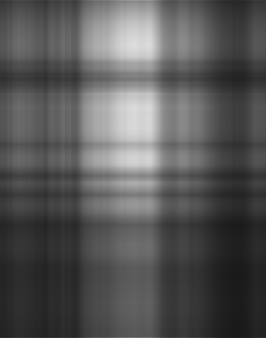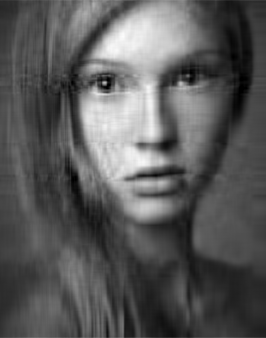可以看到，随着奇异值的增多，图像的特征也逐渐显现出来。
奇异值取前10%时，去噪效果更好：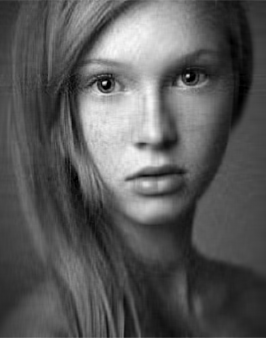这样就实现了对图像简单的降噪处理。
python代码如下：
from numpy import *
from numpy import linalg as la
def svd_denoise(img):
u, sigma, vt = la.svd(img)
h, w = img.shape[:2]
h1 = int(h * 0.1) #取前10%的奇异值重构图像
sigma1 = diag(sigma[:h1],0) #用奇异值生成对角矩阵
u1 = zeros((h,h1), float)
u1[:,:] = u[:,:h1]
vt1 = zeros((h1,w), float)
vt1[:,:] = vt[:h1,:]
return u1.dot(sigma1).dot(vt1)
需要注意的是，linalg中的svd 函数返回u、sigma、yt，而sigma是一个由大到小排列的一维向量，而在后续的处理中需要用numpy中的diag函数将其变成一个对角矩阵。


展开全文图像处理 python
• SVD 图像处理 奇异值分解在图形压缩中的应用 文章目录(1) 奇异值分解(2) 利用 SVD 对原数据进行降维(3) matlab的相关操作1. 对单张图片的处理2. 对文件夹中的图片批量处理3. 视频处理 (1) 奇异值分解 Am×n=Um×m∑m...
SVD 图像处理
奇异值分解在图形压缩中的应用
文章目录(1) 奇异值分解(2) 利用 SVD 对原数据进行降维(3) matlab的相关操作1. 对单张图片的处理2. 对文件夹中的图片批量处理3. 视频处理
(1) 奇异值分解
$A_{m\times n}=U_{m\times m}\sum\nolimits_{m\times n}V^T_{n\times n}$

奇异值矩阵：大小和 $A$ 相同，除了主对角线上元素不为 $0$ 以外，其余位置的元素均为 $0$ 。例如：

$\left[ \begin{matrix} 5&0&0\\ 0&4&0 \end{matrix} \right]$

$U_{m\times m}$ 的求解

计算 $P=A\times A^T$，这是一个 $m$ 阶的对称矩阵。
对 $P$ 进行相似对角化，并按特征值从大到小排列，就可得到 $P=UB_1U^T$。

$V_{n\times n}$ 的求解

计算 $Q=A^T\times A$，这是一个 $n$ 阶的对称矩阵。
对 $Q$ 进行相似对角化，并按特征值从大到小排列，就可得到 $Q=VB_2V^T$。

$\sum\nolimits_{m\times n}$ 的求解

由于 $A\times A^T，A^T\times A$，非零特征值相同。
将这些非零特征值开方并从大到小填入一个和 $A$ 大小相同全零矩阵，形成奇异值矩阵。

(2) 利用 SVD 对原数据进行降维

对原理的直观理解：

首先，一个矩阵是由不同的基组成的，矩阵的基通过一定规律的组合就可以将原矩阵还原，而使用 SVD 方法就是将组成矩阵的基找到，$U_{m\times m}$ 反映的就是找到的基，而保证 $U_{m\times m}$ 是一个正交单位矩阵，是由于正交的矩阵其基的冗余信息最少。
其次，每一个基在原矩阵中的“重要性”不同，而 $\sum\nolimits_{m\times n}$ 就反映这个权重。
$V_{n\times n}$ 是一个记录基的组合方式，进而完成还原的矩阵。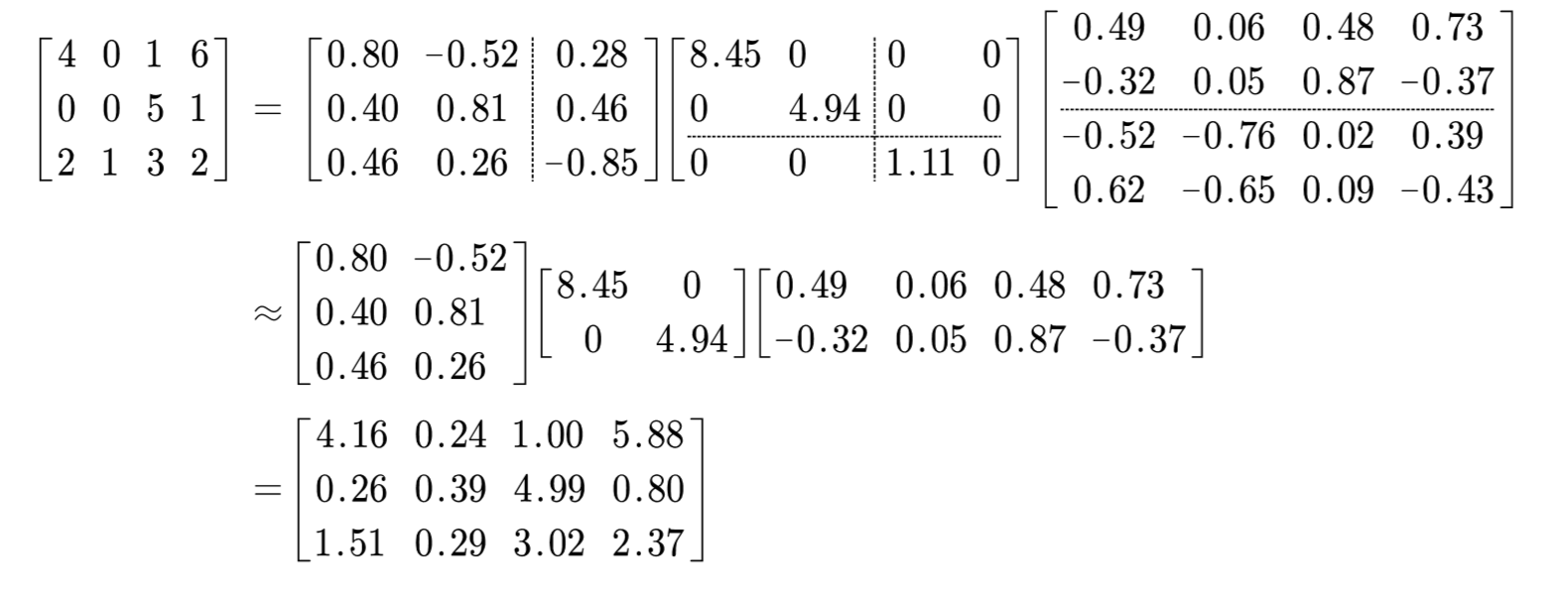通过保留较少的特征值，可以实现降维，注意这里的降维不是表示矩阵的大小减小，而是降低矩阵的秩。
计算保留原矩阵的特征比例$\lambda=\frac{8.45+4.94}{8.45+4.94+1.11}\times 100\%=92.34\%$

(3) matlab的相关操作
1. 对单张图片的处理
$①$ matlab 进行奇异值分解
[U,S,V]=svd(A) ，注意这里的 V 是没有进行过转置的，A 要是一个 $n\times n$ 的矩阵。
$②$ matlab 返回主对角线元素 P=diag(A)
$③$ matlab 读入图片
img=imread(图片地址)，注意这样读出来的是一个 uint8 类型的矩阵，要转化成 double 型的才能进行 SVD 操作。
$④$ matlab 转化为灰色图片 I = rgb2gray(A)
$⑤$ matlab 将 RGB 三个颜色矩阵整合成一个 img=cat(3,r,g,b)
$⑥$ matlab 保存图片
imwrite(uint8(A), 保存地址)，注意这里要把颜色矩阵转化回 uint8。
2. 对文件夹中的图片批量处理
$①$ matlab 将文件名拼接
fullfile('dir1', 'dir2', ..., 'filename')
f = fullfile('dir1', 'dir2', ..., 'filename')
具体例子:
输入：f = fullfile('C:','User','matlab','matlab.m')
得到：f =C:\User\matlab\matlab.m

$②$ matlab 文件结构数组
dirOutput=dir('正则表达式');
dirOutput =

包含以下字段的 struct 数组:

name
folder
date
bytes
isdir
datenum

3. 视频处理
$①$ matlab 读取视频文件
VideoObj=VideoReader(视频文件的地址)

Name - 视频文件名
Path - 视频文件路径
Duration - 视频的总时长（秒）
FrameRate - 视频帧速（帧/秒）
NumberOfFrames - 视频的总帧数
Height - 视频帧的高度
Width - 视频帧的宽度
BitsPerPixel - 视频帧每个像素的数据长度（比特）
VideoFormat - 视频的类型, 如 'RGB24'.
Tag - 视频对象的标识符，默认为空字符串''

$②$ matlab 读取指定帧 video = read(v,index) 只读取 index 指定的帧。


展开全文matlab
• SVD图像压缩步骤 对于RGB图像来说，对应三色层，对每一个色层进行SVD分解，得到U,S,VU, S, VU,S,V矩阵，设利用前K大奇异值进行图像压缩，即有: result=∑i=13Ui[:,1:K]∗S[1:K,1:K]∗VT[1:K,:]result = \sum_{i = 1}...
SVD图像压缩步骤
对于RGB图像来说，对应三色层，对每一个色层进行SVD分解，得到$U, S, V$矩阵，设利用前K大奇异值进行图像压缩，即有:
$result = \sum_{i = 1}^{3}U_i[:, 1:K] * S[1:K, 1:K] * V^T[1:K, :]$
而对于灰度图像来说，只需要完成一层的运算即可。
原图及不同保留度下压缩结果
原图像：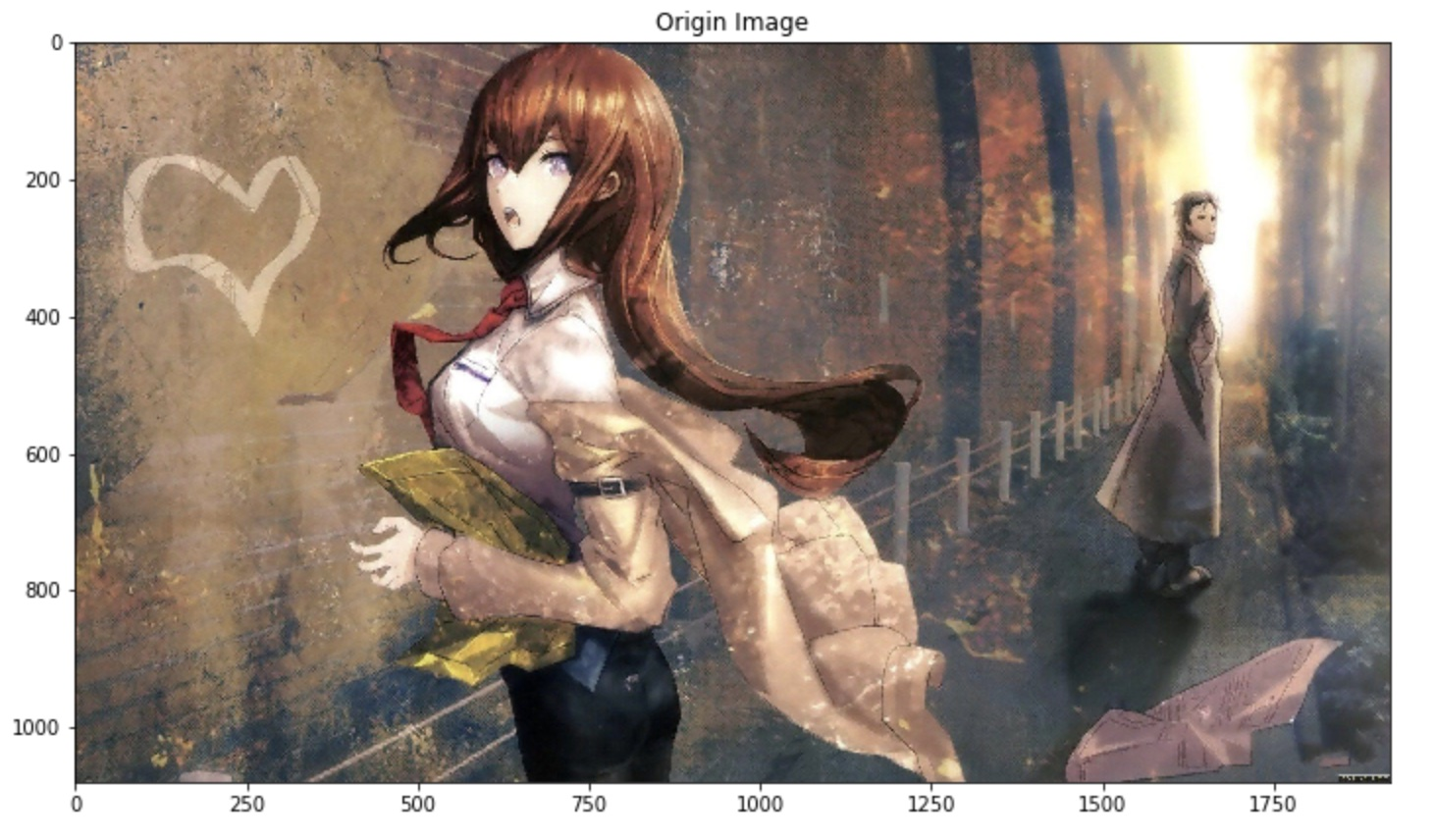不同保留度下的压缩结果：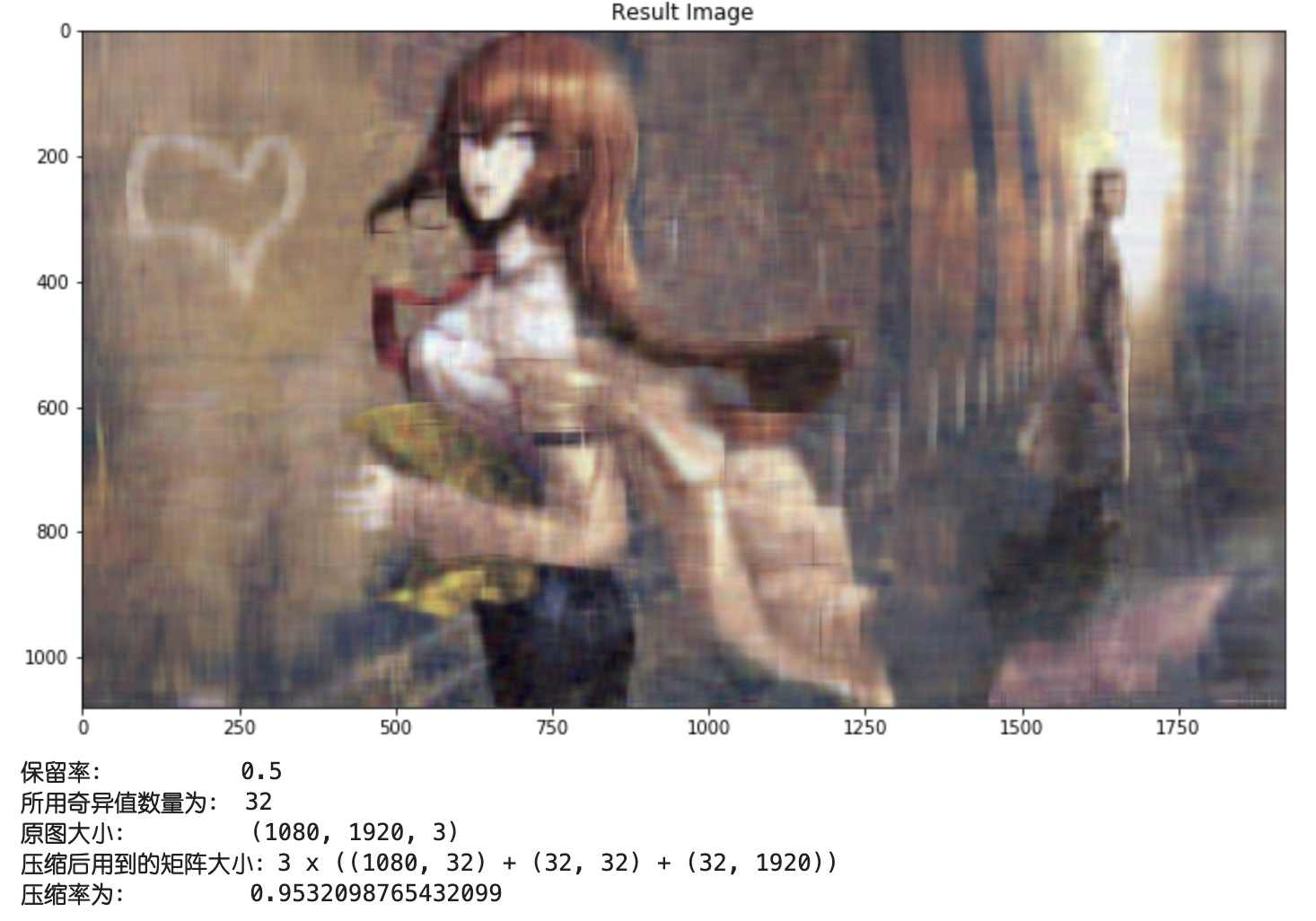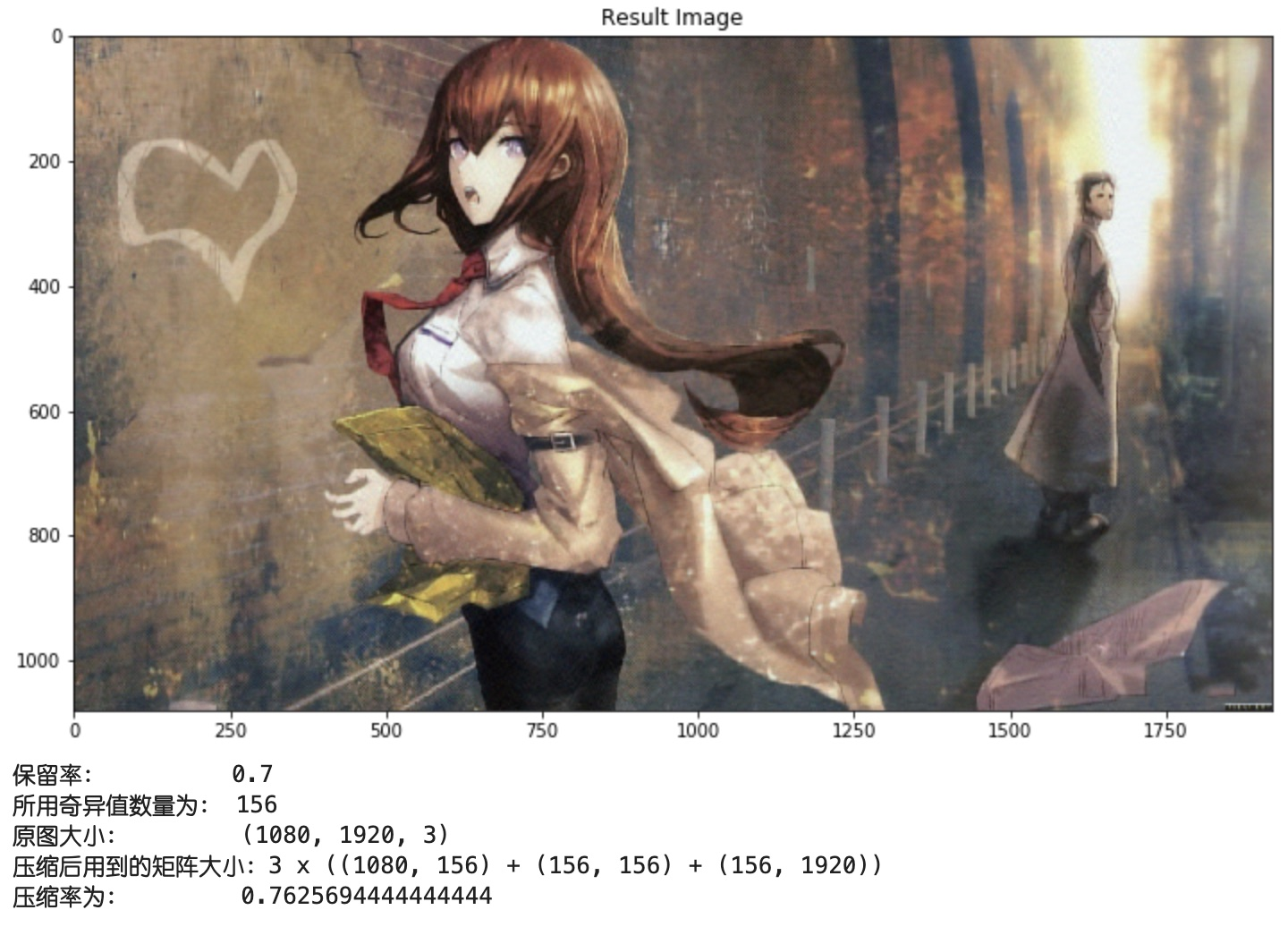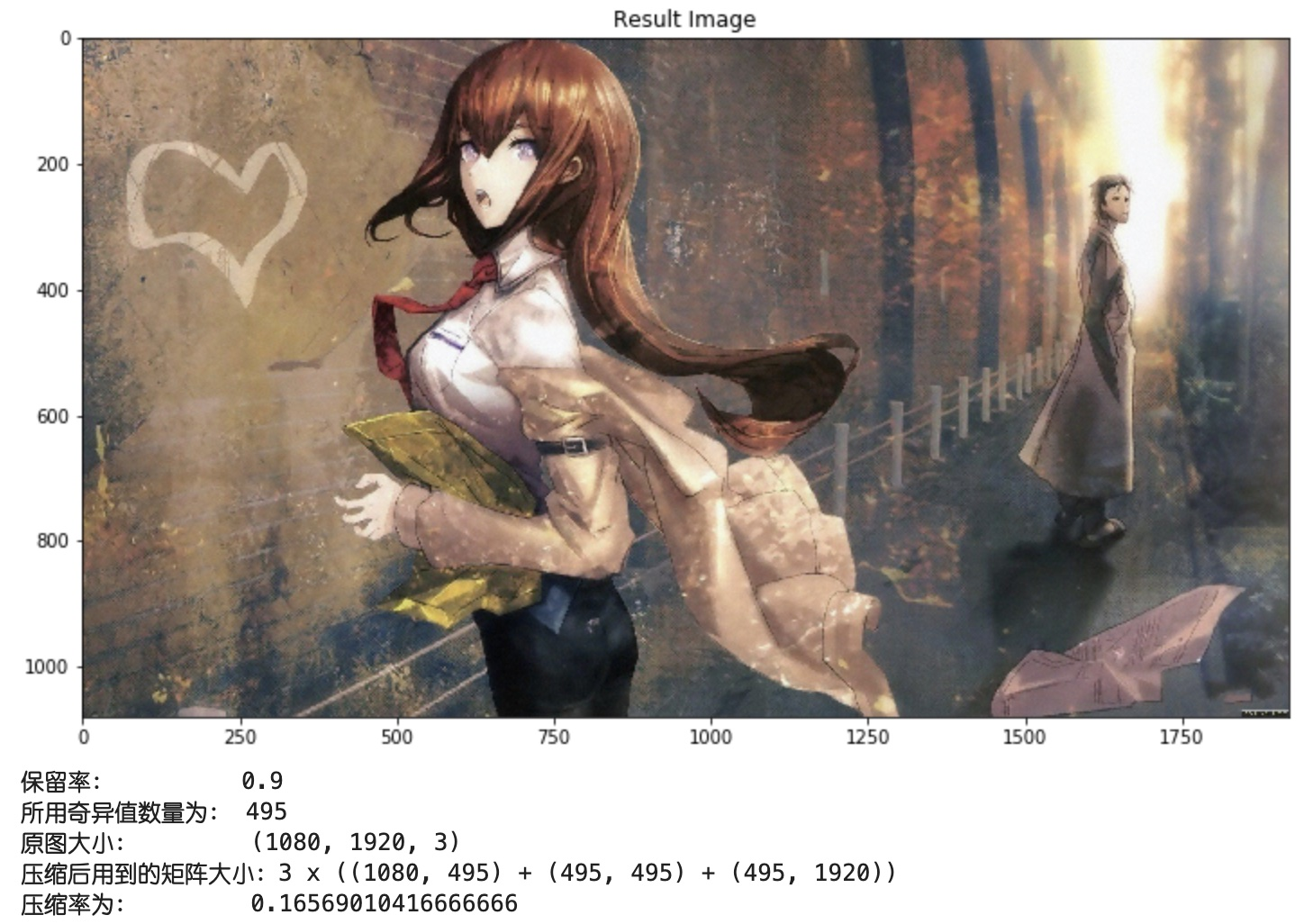Show me the code
导包
import numpy as np
import matplotlib.pyplot as plt

SVD图像压缩模块
def zip_image_by_svd(origin_image, rate = 0.8):
# 显示原图像
plt.figure(figsize= (12, 12))
plt.title("Origin Image")
plt.imshow(origin_image)
plt.show()

print("\n\n======开始压缩======")
# 提前开辟结果存放空间
result = np.zeros(origin_image.shape)

# 对原图像进行SVD分解
u_shape = 0
s_shape = 0
vT_shape = 0

for chan in range(3):
# 对该层进行SVD分解
U, sigma, V = np.linalg.svd(origin_image[:, :, chan])
n_sigmas = 0
temp = 0

# 计算达到保留率需要的奇异值数量
while (temp / np.sum(sigma)) < rate:
temp += sigma[n_sigmas]
n_sigmas += 1

# 构建奇异值矩阵
S = np.zeros((n_sigmas, n_sigmas))

for i in range(n_sigmas):
S[i, i] = sigma[i]

# 构建结果
result[:, :, chan] = (U[:, 0:n_sigmas].dot(S)).dot(V[0:n_sigmas, :])
u_shape = U[:, 0:n_sigmas].shape
s_shape = S.shape
vT_shape = V[0:n_sigmas, :].shape

# 归一化到[0, 1]
for i in range(3):
MAX = np.max(result[:, :, i])
MIN = np.min(result[:, :, i])
result[:, :, i] = (result[:, :, i] - MIN) / (MAX - MIN)

# 调整到[0, 255]
result  = np.round(result * 255).astype('int')

# 显示压缩结果
plt.figure(figsize= (12, 12))
plt.imshow(result)
plt.title("Result Image")
plt.show()

# 计算压缩率
zip_rate =(origin_image.size -3 * (u_shape * u_shape + s_shape * s_shape + vT_shape * vT_shape)) / (origin_image.size)

print("保留率：        ", rate)
print("所用奇异值数量为：", n_sigmas)
print("原图大小：       ", origin_image.shape)
print("压缩后用到的矩阵大小：3 x ({} + {} + {})". format(u_shape, s_shape, vT_shape))
print("压缩率为：       ", zip_rate)

主函数及其调用
# 定义主函数
def main():
# 读入图像
image_path = '/Users/tantaiyunfei/Desktop/图片/背景图/e32e0ab9393fb231aa3af9a71ac06a07.jpg'

# 利用自定义SVD图像压缩模块对图像进行压缩
zip_image_by_svd(origin_image, rate = 0.8)

# 主函数调用
if __name__ == "__main__":
main()



展开全文图像压缩
•   利用SVD是可以对图像进行压缩的，其核心原因在于，图像的像素之间具有高度的相关性。 代码 # -*- coding: utf-8 -*- ''' author@cclplus date:2019/11/3 ''' import cv2 from PIL import Image import ...
前言
SVD即奇异值分解（singular value decomposition），是将矩阵分解为奇异向量和奇异值。
每次写相关文章的时候，都会面临一个常见的问题，矩阵是什么？套用平冈和幸的话来说，矩阵即是映射，令矩阵y=Ax,那么矩阵A就是由向量x到向量y的一个映射。从另一个角度看，矩阵又是数的排列。SVD的核心思想是，通过较数据量较少的多个矩阵的映射去还原一个数据量更大的数的排列。即只要A,x两者的数字数量相加小于y，我们就实现了数据压缩。对于图像的压缩，可以通过PSNR进行判断。
SVD的用处之一，即是用于图像的数据压缩。因为实际图像各像素点之间具有高度相关性，因此，使用更少的像素取表示整张图像的信息是可行的。
代码
# -*- coding: utf-8 -*-
'''
author@cclplus
date:2019/11/3
'''
import cv2
import matplotlib as mpl
import numpy as np
import matplotlib.pyplot as plt
#转为u8类型
def restore1(u, sigma, v, k):
m = len(u)
n = len(v)
a = np.zeros((m, n))
a = np.dot(u[:, :k], np.diag(sigma[:k])).dot(v[:k, :])
a[a < 0] = 0
a[a > 255] = 255
return np.rint(a).astype('uint8')
def SVD(frame,K=10):
a = np.array(frame)
#由于是彩色图像，所以3通道。a的最内层数组为三个数，分别表示RGB，用来表示一个像素
u_r, sigma_r, v_r = np.linalg.svd(a[:, :, 0])
u_g, sigma_g, v_g = np.linalg.svd(a[:, :, 1])
u_b, sigma_b, v_b = np.linalg.svd(a[:, :, 2])
R = restore1(u_r, sigma_r, v_r, K)
G = restore1(u_g, sigma_g, v_g, K)
B = restore1(u_b, sigma_b, v_b, K)
I = np.stack((R, G, B), axis = 2)
return I

if __name__ == "__main__":
mpl.rcParams['font.sans-serif'] = [u'simHei']
mpl.rcParams['axes.unicode_minus'] = False
I = SVD(frame,40)
plt.imshow(I)
cv2.imwrite("out.bmp",I)

原图取二十个特征值展开全文python SVD
• 人脸识别可以说是当今社会十分热门而且应用广泛的一项技术，应用于案件侦破、设备解锁、网络信息安全等多领域...有关R的部分可以看我另外一篇文章(基于R语言的SVD图像处理）我在本篇文章中使用三种方法：线性回归最...
人脸识别可以说是当今社会十分热门而且应用广泛的一项技术，应用于案件侦破、设备解锁、网络信息安全等多领域。在图像识别中一个最大的难点在于图像的数据量很大，如何充分地利用信息。不同的照片具有不同的像素和比例，本文用 python 进行图片像素处理，R 语言做 RGB 格式向灰度图片的转换以及SVD分解。有关R的部分可以看我另外一篇文章(基于R语言的SVD图像处理）我在本篇文章中使用三种方法：线性回归最小残差法、logistic 回归、最短欧几里德距离法进行人脸识别性别判定。
思路概述
首先收集挑选训练集，尽量选取和测试集相一致的训练集， 然后基于训练集和测试集的不同照片的像素尺寸的不同，必须进行处理。另外，因 RGB 式彩色图片是高维数组，考虑如何处理为矩阵以方便数据分析。
其次要区别目标图片是男是女，很容易想到聚类或者说分类，按照一定方式将训练集按男女聚在一起，计算测试集和训练集之间的某个量来区别是男是女。如果采用欧几里德距离，即欧几里德最短距离法，也可以采用线性回归最小残差来区分属于哪一类。另外，男女为两分类变量，引入虚拟变量 0/1 代表男女，进行 logistic 回归也可预测结果。
另外，因为图像数据为超高维矩阵，无法直接应用于这些方法，而且会出现过拟合和效率低下甚至处理器难以计算的情况，因此必须进行数据降维来提取关键信息进行算法实现，我采用奇异值分解进行数据处理，选取适当的奇异值(奇异向量) 来进行实现。
图像初步处理
首先需要将你的训练集以及样本集处理为相同像素的图片，可是使用如下python代码：
from PIL import Image

def produceImage(file_in, width, height, file_out):
image = Image.open(file_in)
resized_image = image.resize((width, height), Image.ANTIALIAS)
resized_image.save(file_out)

if __name__ == '__main__':
file_in = '/Users/tyc_219/Desktop/tex/test_%03d.jpg'
width = 200
height = 200
file_out = '/Users/tyc_219/Desktop/training/sam_%03d.jpg'
produceImage(file_in, width, height, file_out)

其中的width以及height你可以自己指定，我在这里指定的大小是200*200像素。
加权值公式为:
$Gray = 0.299 ∗ R + 0.587 ∗ G + 0.114 ∗ B$
图像像素处理和灰度转换应用于所有图片。
算法
线性回归残差法
线性回归的残差平方和大小反映了模型拟合的程度，反映了变量之间的相关程度，如果测试对象为男性，则以该测试对象为因变量，以训练集中男性数据为因变量，则模型应有较好的拟合，相反，如果以女性训练集为因变量进行拟合，拟合效果将不如前者。 因此我们对每一个测试对象，分别以男女训练集进行线性拟合，比较模型拟合结果的残差平方和，根据最小残差平方和将其分类为男或女。
但是以男女训练集为因变量，维度过高，变量个数较多，造成拟合效率不高，甚至过拟合现象。故使用奇异值分解，对训练集图像进行矩阵近似，选择关键信息，选择部分较大奇异值对应的 U 矩阵中的奇异向量进行拟合。
一般来说：当选择 10～20 个较大奇异值对应的奇异向量进行拟合并判定时，可以得到很高的准确率
Logistic 回归
因为我们要估计的目标是性别，即二元变量，那么我们就可以使用 logistic 回归进行拟合，并用拟合结果估计测试集的性别。
同样，因为测试集和训练集数据量较大，我们需要进行奇异值分解。这里将训练集和测试集合并再进行奇异值分解，这样，选择适当个数的分解后前几个奇异值对应的奇异向量的个数进行拟合，再选择测试集对应的相应个数的奇异向量进行估计。
欧几里德距离法
我们可以对训练集根据性别不同进行一个简单平均，即可得到平均脸，通过平均脸可以观察到不同性别所显示出来的面部特征，欧几里德最短距离法就是基于平均脸而进行判别，我们已经得到男女平均脸，即两个类，那么我们就可以跟据样本与两个类之间的距离来将样本分类，这里我们采用欧几里德距离。即计算测试集与男女平均脸矩阵向量之间的距离来将测试集的所有样本分类。
R代码
下面给出供大家参考的R代码，如有不足，大家可以自行改进。
rm(list = ls())
library(RSpectra)
library(jpeg)
library(animation)
#设置读取路径
setwd("/Users/tyc_219/Desktop/training")
png("new_pic%03d.png")
r <- 0.299
g <- 0.587
b <- 0.114
#读取图片，训练集是200张图片
for(i in 1:200)
{
R <- pic[,,1]
G <- pic[,,2]
B <- pic[,,3]
new_pic <- r*R + g*G + b*B   #通过灰度进行图像转化
#将转化后的灰度图导出为.jpg
writeJPEG(new_pic, sprintf("new_pic%03d.jpg", i))
}
dev.off()

train <- matrix(0, nrow = 200, ncol = 40000)   #创建训练集矩阵，每一行为一张图片
for(j in 1:200)
{
train[j,] <- as.vector(t(ma))
}
str(train)

#创建训练集性别矩阵，1代表男性，0代表女性
sex <- c(1,1,1,0,1,0,1,0,1,0,1,1,1,0,1,1,1,0,0,1,1,1,0,1,1,0,0,0,0,1,0,1,1,
1,1,0,0,1,1,1,1,0,0,1,0,1,1,1,1,1,0,1,1,0,1,1,1,0,0,0,0,1,1,1,0,0,1,1,1,1,0,
1,0,1,1,1,1,1,1,1,1,1,1,0,1,0,1,1,1,1,1,1,1,1,1,1,1,1,0,0,1,0,1,1,1,1,
0,0,1,1,1,1,1,1,1,0,1,1,1,1,0,1,1,1,1,1,0,0,0,1,0,0,0,1,1,0,1,0,0,1,0,0,1,0,1,0,
0,0,1,0,1,0,1,0,0,1,0,0,1,0,0,0,1,0,1,1,0,0,1,1,1,1,0,1,1,0,0,0,0,0,
1,1,1,1,0,1,1,0,1,1,1,1,1,0,0,1,1,1,1,1)
sex <- matrix(sex, nrow = 1)

male_female <- 0:1
pic_mean <- matrix(0, 40000, 2)
for (k in male_female) {
index <- (k == sex)
imgi <- train[index, , drop = FALSE] #定位男女在训练样本图像矩阵的行，并提取出来
imgi.mean <- colMeans(imgi) #求该性别对应的训练集在各行的平均值，作为该性别的对比值
pic_mean[,k + 1] <- imgi.mean   #将各性别的图像矩阵（平均值）放入pic_mean矩阵中
}

par(mfrow = c(1, 2)) #画出基于训练集的男女平均脸
for (i in 1:2) {
image(matrix(pic_mean[, i], ncol = 200)[,200:1], col = gray(0:255/255))
}

#使用不同的性别基础进行最小二乘法并找到最小残差，最小残差对应的性别为判别性别
test_img <- matrix(0, nrow = 23, ncol = 40000)
for(j in 1:23)
{
test_img[j,] <- as.vector(t(ma))
}

image_recognition <- function(test)
{
resid.norm <- matrix(NA, 2, 1, dimnames = list(0:1, "resid"))
#对于男女分别进行lm拟合
for (i in 0:1) {
img.mat <- t(train[i == sex, , drop = FALSE])
img.matSVD <- svd(img.mat)
basis.max <- 30
resid.norm[i+1, ] <- norm(matrix(lm(test ~ 0 +
img.matSVD$u[, 1:basis.max])$resid), "F")
}
#将残差最小对应的性别取出作为判别的性别
rec_sex <- match(min(resid.norm), resid.norm)
return(rec_sex-1)
}

sex_test <- vector()
name <- c()     #自行加入名称
system.time(for(m in 1:23)
{
sex_test[m] <- image_recognition(test_img[m,])
if(sex_test[m] == 1) sex_test[m] <- "男"
if(sex_test[m] == 0) sex_test[m] <- "女"
})
cbind(name,sex_test)

logis <- glm(t(sex) ~ ., data = data1, family = "binomial")
summary(logis)

train.svd <- svd(train)
str(train.svd)
u <- train.svd$u par(mfrow = c(1,2)) plot(1:length(train.svd$d), train.svd$d, xlab="i-th sigma", ylab="sigma", main="Singular Values") plot(1:length(train.svd$d), cumsum(train.svd$d)/sum(train.svd$d), main="Cumulative Percent of Total Sigmas")
data1 <- as.data.frame(u[,1:25])

sex.true <- c(1,0,0,1,1,1,1,0,1,0,1,1,0,1,1,1,1,1,1,1,1,1,1)

#计算两个矩阵的欧几里德距离
ec.distance <- function(X, Y) {
dim.X <- dim(X)
dim.Y <- dim(Y)
sum.X <- matrix(rowSums(X^2), dim.X, dim.Y)
sum.Y <- matrix(rowSums(Y^2), dim.X, dim.Y, byrow = TRUE)
dist0 <- sum.X + sum.Y - 2 * tcrossprod(X, Y)
out <- sqrt(dist0)
return(out)
}

#对于未知性别的人脸图像，比较它与男女图像平均值的距离
test.sample <- 1:23

#计算测试集到训练集平均值的距离
system.time(test.distance <- ec.distance(test_img[test.sample, ], t(pic_mean)))
rec.result <- apply(test.distance, 1, which.min) - 1
rate <- length(rec.result[sex.true == rec.result])/23;rate

#将训练集与测试集合并后进行SVD分解，矩阵降维后再进行logistic回归拟合
pics <- rbind(train, test_img)
pics.svd.u <- svd(pics)$u train.svd.u <- pics.svd.u[1:200,] #取出训练集对应的u矩阵部分 x <- cbind(rep(1,23),pics.svd.u[200:223,]) rec <- matrix(0, 20, 1) system.time(for (i in 1:20) { #拟合logistic回归，看在取u矩阵不同的列数时判别率的高低 logistic.fit <- glm(t(sex) ~ train.svd.u[,1:i], family = binomial) beta <- matrix(logistic.fit$coefficients, 1)
ind <- i + 1
X <- t(x)[1:ind,]    #x矩阵中第一列为1，对应beta0
respond <- beta %*% X
p <- exp(respond)/(1+exp(respond))
c1 <- p[sex.true == 0] < 0.5    #c1为判别性别为女性与测试集中对应的人人数
c2 <- p[sex.true == 1] >= 0.5   #c2为判别性别为男性与测试集中对应的人人数
rec[i,] <- (sum(c1)+sum(c2))/23    #(c1 + c2)/23为判别正确率
})
t(rec)

————————————————未经允许，请勿转载——————————————————


展开全文R语言 人脸识别 图像识别 SVD 图像处理
•  利用SVD我们能用小的多的数据来表示原始的数据集。这样做，实际上是去除了噪声和冗余信息。我们是从数据中抽取信息。基于这个视角，我们可以把SVD看成从噪声数据中提取相关特征。  先来学习SVD是如何通过隐性...机器学习实战 SVD
• 实现对图片进行SVD 之前在学习截断式SVD的时候, 不知道为什么截断式SVD后能够近似原矩阵? 或者说奇异值究竟有什么含义? 看了以下知乎的答案后, 有了很形象的理解 奇异值的物理意义是什么？ - 郑宁的回答 - 知乎 ...SVD python
• 奇异值分解在图像处理中有着重要应用。假定一幅图像有n∗nn*n个像素，如果将这n2n^2个数据一起传送，往往会显得数据量很大。因此我们希望能够改传送另外一些比较少的数据，在接收端利用这些数据重构原图像。 假定对...矩阵分解 图像压缩 SVD
• 利用SVD实现,我们能够用小得多的数据集来表示原始数据集。这样做,实际上是去除了噪声和冗余信息。简而言之，SVD是一种从大量数据中提取主要关键数据的方法。下面介绍几种应用场景： 1、隐性语义索引 最早的SVD应用...机器学习 数据
• def printMat(inMat, thresh=0.7): for i in range(32): for k in range(32): if float(inMat[i,k]) &gt; thresh: print (1,end=' ') else: print ...SVD方法
• 利用SVD能够实现用小得多的数据集来表示原始数据集，这样做，实际上是去除了噪声和冗余信息。当我们视图节省空间时，去除噪声和冗余信息是目标，但是我们这里则是从数据中抽取信息，基于这个视角，我们可以把SVD看成...SVD降维 奇异值分解 推荐系统 协同过滤
• 关于PCA和SVD的区别，以及简单的应用实例简述SVD和PCA    PCA    SVD    区别功能快捷键合理的创建标题，有助于目录的生成如何改变文本的样式插入链接与图片如何插入一段漂亮的代码片生成一个适合你的列表创建...机器学习 特征提取 matlab 矩阵降维
• 奇异值分解在图像处理中有着重要应用。假定一幅图像有n∗n个像素，如果将这n^2个数据一起传送，往往会显得数据量很大。因此我们希望能够改传送另外一些比较少的数据，在接收端利用这些数据重构原图像。 假定对矩阵A...
• 在很多情况下，数据的绝大部分信息往往集中在很小一部分数据上，我们知道线性代数中有很多矩阵的分解技术可以将矩阵表示成易于处理或是表达简化的形式。最常见的一就种是SVD(Singular Value Decomposition)算法。SVD
• 感谢以下2位的渊博知识：（1）李春春：http://blog.csdn.net/zhongkelee/article/details/44064401（2）沈春旭： https://blog.csdn.net/shenziheng1/article/details/52916278一、PCA简介1. 相关背景 上完陈恩红...图像处理 PCA SVD
• 利用SVD分解压缩图像 利用SVD分解求超定方程的解 直接对矩阵进行奇异值分解 已知矩阵，对其进行奇异值分解。 import numpy as np #创建矩阵A A = np.array([[1,5,7,6,1],[2,1,10,4,4],[3,6,7,5,2]]) #利用np....奇异值分解 图片压缩 超定方程求解 矩阵分解
• 利用SVD提升推荐引擎的性能 餐馆可分为很多类别，不同的专家对其分类可能有不同依据。实际中，我们可以忘掉专家，从数据着手，可对记录用户关于餐馆观点的数据进行处理，并从中提取出其背后的因素。这些因素可能会与...机器学习
• 利用SVD（Singular Value Decomposition），即奇异值分解，我们可以用更小的数据集来表示原始数据集。这样做，其实是去除了噪声和冗余信息。机器学习 数据 算法
• SVD目录一、前言二、SVD的应用1、隐性语义索引2、推荐系统三、SVD的原理1、特征值分解2、奇异值分解四、利用python实现SVD1、基于协同过滤的...餐馆菜肴推荐引擎1、推荐未尝过的菜肴2、利用SVD提高推荐的效果六、示例...学习笔记
• 协方差矩阵的特征向量为数据传播的方向，特征值为该方向上的幅度。 第二大特征向量正交于最大特征向量，指向第二大数据传播方向。因为只有方阵才能求其特征值与特征向量，所以非方阵Data就只先转化为方阵：Data * ...
...